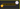# How to implement Quicksort algorithm in JavaScriptIn this tutorial, we are going to learn about quicksort algorithm and its implementation in JavaScript.

## Quicksort

• it is an efficient sorting algorithm for the larger datasets where it performs faster than the merge sort and heap sort.
• It was Developed by a British computer scientist Tony Hoare in 1959 and published in 1961.

## How does quicksort works?

Consider an array which has 5 items `[3,6,4,1,2]`

1. First, we need to choose the pivot value from the array, we can choose any thing as a pivot value so that we are choosing last item `2` as a pivot.
2. Next, we need to loop through the array and compare with pivot value `2`. If any item which is less than pivot value place that on left-hand side else if any item is greater than the pivot value place that item on the right-hand side.1. We need to run the quickSort recursively on right part and left part to get the sorted array.

## Quicksort algorithm implementation

Let’s implement the quicksort algorithm in JavaScript.

First, we are creating a `quickSort` function with three parameters which are `arr`, `length` and `start`.

arr : unsorted array.

length : How many times we need to loop.

start: loop starting point.

``````function quickSort(arr,length = arr.length-1,start=0){

if(arr.length < 2){
return arr; // base case
}
const pivot = arr[arr.length-1];
const left  = [ ];
const right = [ ];
}``````

Next, we are using a while loop which helps us to loop over the items in a unsorted array, if any item is less than pivot value push that item into the left array else push into the right array.

``````function quickSort(arr,length = arr.length-1,start=0){

if(arr.length < 2) {
return arr;
}
const pivot = arr[arr.length-1];
const left  = [ ];
const right = [ ];

while (start < length) {
if (arr[start] < pivot){
left.push(arr[start])
}
else {
right.push(arr[start])
}
start++ //  incrementing start value
}
}``````

The final step we need to run the quickSort recursively on both left-hand side and right-hand side to get the sorted array.

``````function quickSort(arr, length = arr.length - 1, start = 0) {

if (arr.length < 2) return arr // base case

const pivot = arr[arr.length - 1]; //pivot value
const left = [ ];  // left handside array
const right = [ ]; // right handside array

while (start < length) {  // comparing and pushing
if (arr[start] < pivot){
left.push(arr[start])
}
else {
right.push(arr[start])
}
start++ //  incrementing start value
}
// calling quick sort recursively
return [...quickSort(left), pivot, ...quickSort(right)];
}

console.log(quickSort([4, 9, 2, 6, 8, 10, 3, 1, 7, 5]))

//output => [1, 2, 3, 4, 5, 6, 7, 8, 9, 10]``````

In the above code we used spread operator to combine the left and right part of the arrays.

## Quicksort Time complexity

• Average case - O(nlogn)
• Best case - O(nlogn)

## Top Udemy Courses##### JavaScript - The Complete Guide 2020 (Beginner + Advanced)
45,614 students enrolled
52 hours of video content
View Course##### React - The Complete Guide (incl Hooks, React Router, Redux)
284,472 students enrolled
40 hours of video content
View Course##### Vue - The Complete Guide (w/ Router, Vuex, Composition API)
130,921 students enrolled
48.5 hours of video content
View Course